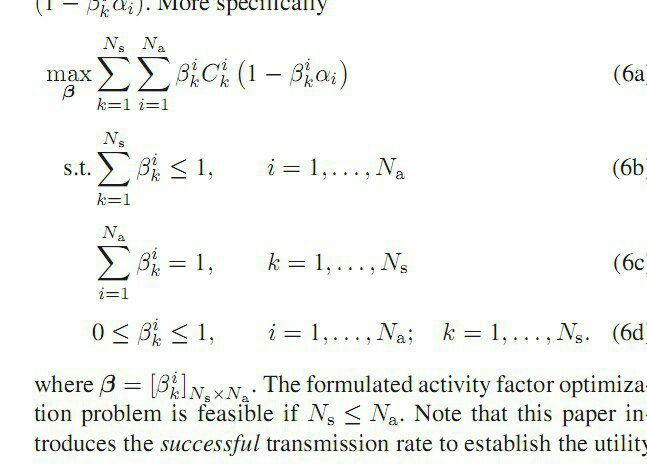# Why I don't get the optimum point?

hello,
why I don’t get the optimum point?
thanks
clc
clear all
alpha=.1;
c=3;s=0;
p=3;
cvx_begin sdp
variable b(p, p)
expression f2

for k=1:p
for i=1:p
s=s+1;
fg=b(k,i)c;
f2(s) =fg
(1-(b(k,i)alpha));
end
end
maximize sum(f2)
subject to
for ii=1:p
sum(b(ii,:),1)==1;
sum(b(:,ii),1)<=1;
end
zeros(p
p,1)<=vec(b)<=ones(p*p,1);
cvx_endYou specified an infeasible problem, which was reported as such by CVX.

Inside the for loop.
`sum(b(ii,:),1)==1;`
constrains every element of b to =1 (a row vector at a time); and
`sum(b(:,ii),1)<=1;`
constrains all column sums of b to be <= 1. So this is of course infeasible given that b has more than one row.

I think what you want is, with no for loop,

``````sum(b,2) == 1
sum(b,1) <= 1
``````

or perhaps

``````sum(b,1) == 1
sum(b,2) <= 1
``````

I’ll let you figure out which is correct.

On the problem you provided, both versions had the solution `b = 1/3*ones(3)`.

Given that you have no semidefinite constraints, you can avoid a potential source of error by not using the sdp option with cvx_begin.

1 Like

Thanks for your help. But is it meaningful that optimum solution is the same?

I believe that occurs because your c and alpha values are scalars, making the problem invariant to transposition of b. That invariance may not hold for general c and alpha, as in the image.